# Fractional Factorial Designs with SigmaXL

### What Are Fractional Factorial Designs with SigmaXL?

In simple terms, a fractional factorial design with SigmaXL is a subset of a full factorial experiment.

• Fractional factorials use fewer treatment combinations and runs.
• Fractional factorials are less able to determine effects because of fewer degrees of freedom available to evaluate higher order interactions.
• Fractional factorials can be used to screen a larger number of factors.
• Fractional factorials can also be used for optimization.

### Why Fractional Factorial Experiments?

To run a full factorial experiment for k factors, we need 2k unique treatments. In other words, we need resources that can afford at least 2k runs.

With k increasing, the number of runs required in full factorial experiments rises dramatically even without any replications, and the percentage of degrees of freedom spent on the main effects decreases. However, the higher order interactions (3 or 4 factor interactions) can typically be ignored, which allows us to run fewer trials to understand the main effects and two-way interactions.

The main effects and two-way interaction are the key effects we need to evaluate. The higher order the interaction is, the more we can ignore it.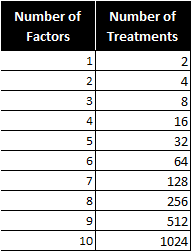Notice the number of treatments increases dramatically as factors are added.

### How Does a Fractional Factorial Work?

We are trying to find the cause-and-effect relationship between a response (Y) and three factors (factor A, B, and C) and their interactions (AB, BC, AC, and ABC). As follows is the 23 full factorial design (2 level 3 factor). There are eight treatment combinations (2 * 2 * 2).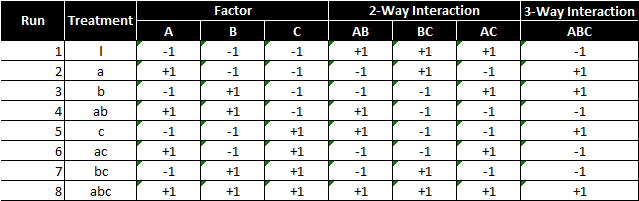To perform a 23 full factorial experiment, we need to run at least eight unique treatments (2 * 2 * 2).
What if we only have enough resources to run four treatments?
As a result, we need to carefully select a subset from the eight treatments so that all of our main effects can be evaluated and the design can be kept balanced and orthogonal.

#### Example of an invalid design

This design is invalid because only the low setting of factor C is tested. We cannot evaluate the main effect of factor C using this design. Remember orthogonality?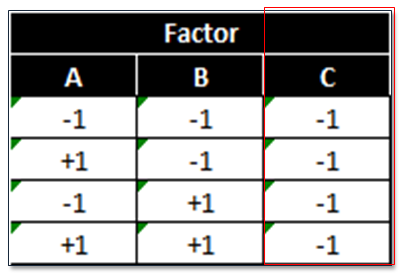This design is also invalid because it is neither balanced nor orthogonal. Checking orthogonality: the sum of AC interaction signs should equal zero (0).

• Run 1 (−)
• Run 2 (−)
• Run 3 (−)
• Run 4 (+)
• Sum (−1)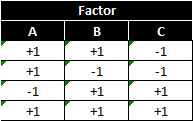This design has a low and high setting for each factor, but is not orthogonal. To select the four treatments run in the 23−1 fractional factorial experiment, we start from the 22 full factorial design of experiment. If we replace the two-way interaction (AB) column with the factor C column, the design will be valid.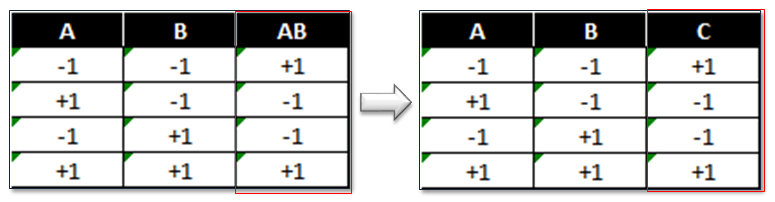Imagine a two-factor full factorial with factors A and B. We also learn about the interaction of A and B. In a fractional factorial, we sacrifice learning about the two-way interaction between A and B, and substitute factor C.

### 23−1 Fractional Factorial Design Pattern

This pattern implies three factors and four treatments.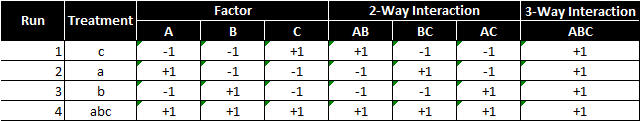Note: We also call this kind of design a half-factorial design since we only have half of the treatments that we would have in a full factorial design. In 23−1 fractional factorial design of experiment, the effect of three-way interaction (ABC) is not measurable since it only has “+1”.
In four runs, we are able to run high and low settings for each of the three factors. The three-way interaction ABC is only at the high setting. In the 23−1 fractional factorial design, we notice that the column of each main effect has identical “+1” and “−1” values with one two-way interaction column.

• A and BC
• B and AC
• C and AB

In this situation, we say that A is aliased with BC or A is the alias of BC. By multiplying any column with itself, we obtain the identity (I).

A*A=I

The product of any column and the identity is the column itself.

A*I=A

Column ABC is called the generator. By multiplying any column with the generator, we obtain its alias.

A*ABC=(A*A)*BC=I*BC=BC

### Fractional Factorial Designs with SigmaXL (Experiments)

Step 1: Initiate the experiment design

1. Click SigmaXL -> Designs of Experiments -> 2-Level Factorial/Screening -> 2-Level Factorial/Screening Designs
2. A window named “2-Level Factorial/Screening Designs of Experiments” pops up.

Step 2: Enter the response and factors information in the window “2-Level Factorial/Screening Design of Experiments”

1. One response: Y
2. Three factors: A, B and C
3. Two-level design: each factor has two settings
4. Select “4-Run, 2**(3-1), ½ Fraction, Res III”.
5. Select the number of replications and make design, we will assume there is sufficient resources allow each treatment to be run twice. Enter “2” into the box of “Number of Replicates”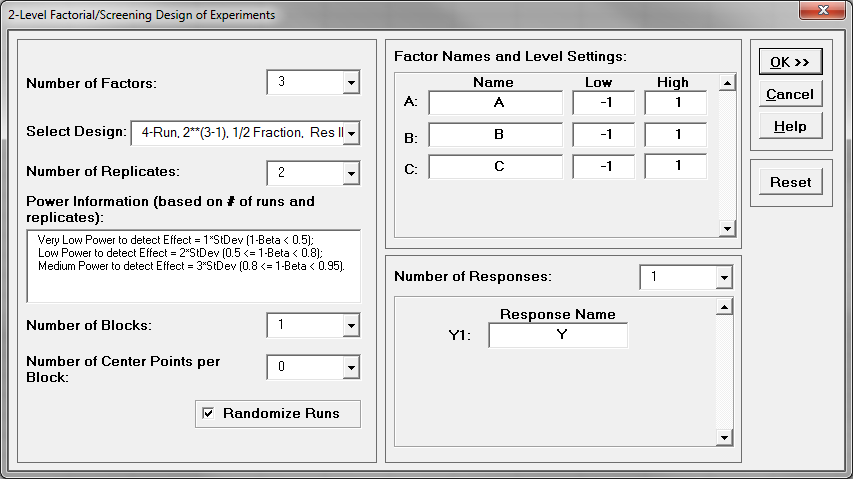6. Click “OK>>”
7. The DOE template appears in the newly generated tab “3 Factor DOE”.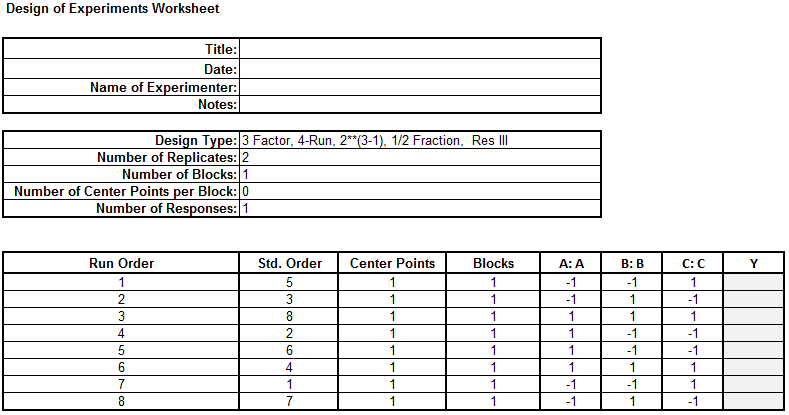Step 3: Implement the experiment and record the results in cell labeled “Y” in the DOE table. The data has been provided for you in the DOE Fractional data table in your Sample Data.xlsx file. Carefully (paying close attention to using the “A” and “B” and “C” settings to map your “Y” results) enter the “Y” values into your newly generated DOE template.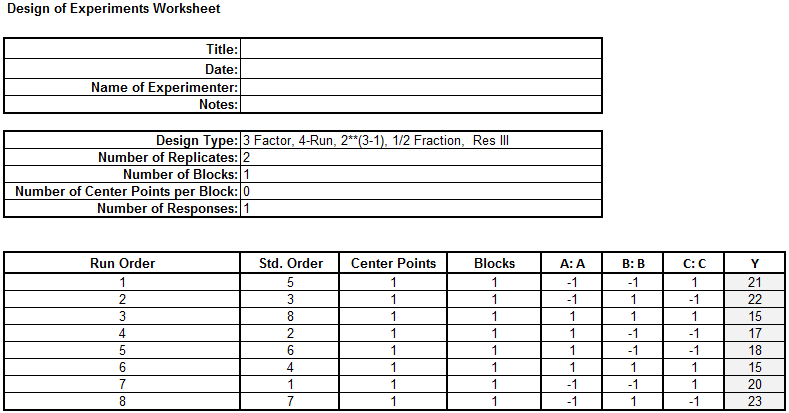Step 4: Fit the model using the experiment results

1. Click SigmaXL -> Design of Experiments -> 2-Level Factorial/Screening -> Analyze 2-Level Factorial/Screening Design
2. A new window named “Analyze 2-Level Factorial/Screening Design” appears with the response and factors pre-populated.
3. Check the checkbox “Show Residual Plots”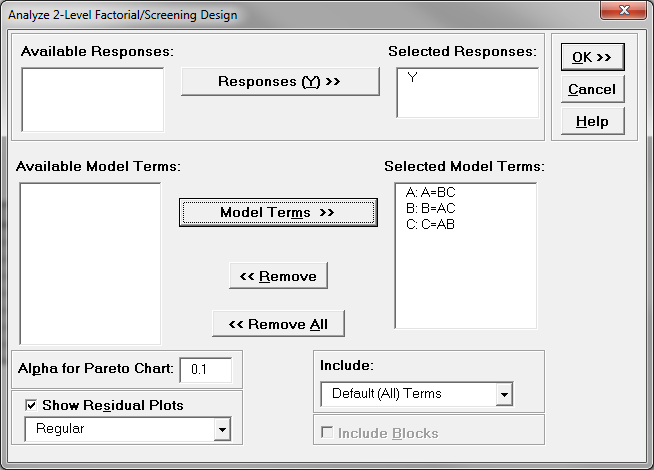4. Click “OK>>”

Step 5: Analyze the model results

1. Check whether the model is statistically significant.
2. Check which factors are insignificant
3. If any independent variables are not significant, remove them one at a time and rerun the model until all the independent variables in the model are significant.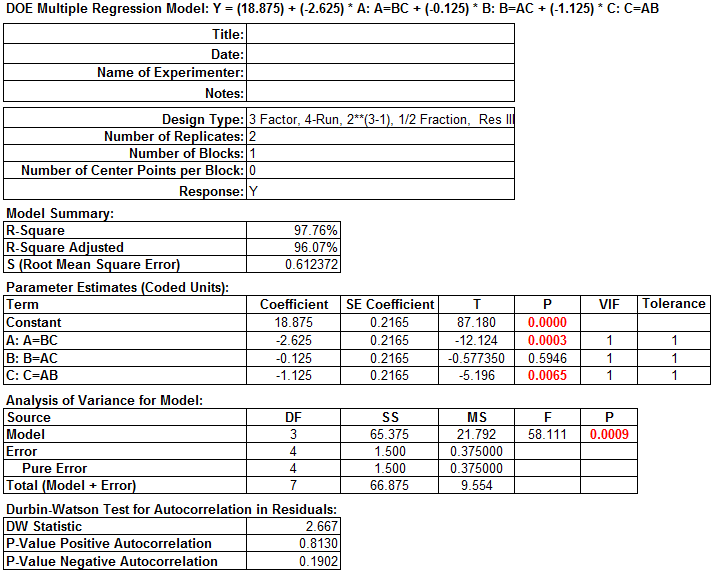The p-value of factor B is greater than the alpha level (0.05), so it is not statistically significant. In this example, since factor B is not statistically significant, it needs to be removed from the model.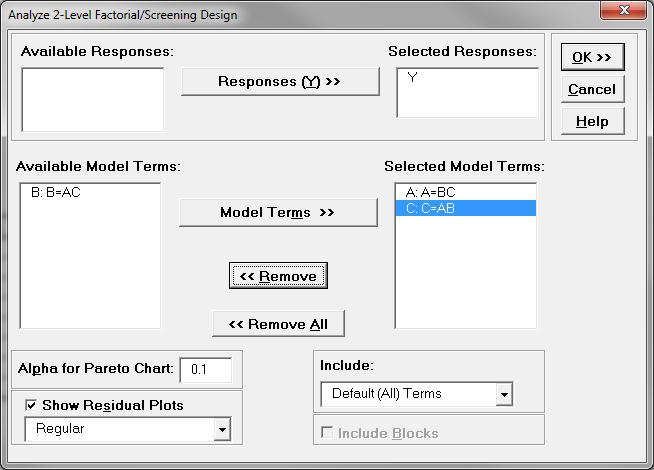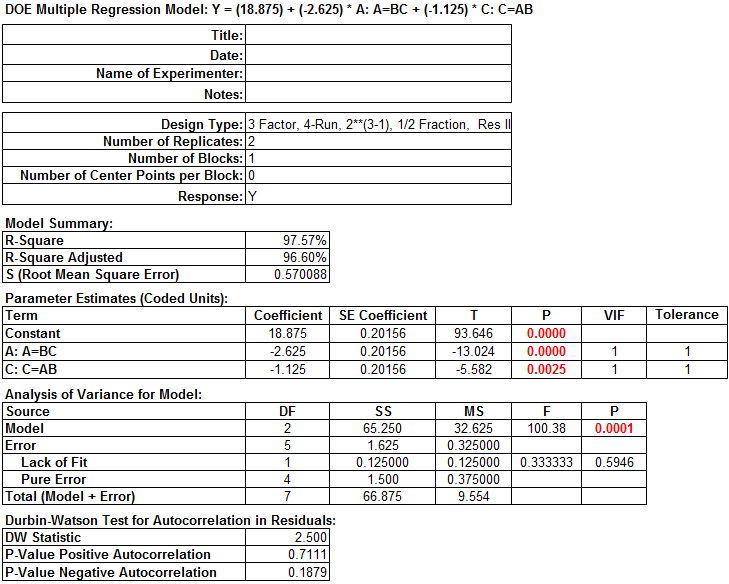The p-values of all the independent variables are smaller than 0.05. There is no need to remove any independent variables from the model.

Step 6: Conduct residual analysis to ensure that the residuals of the model satisfy the following criteria. Because you already elected to check the box to “Show Residual Plots”, the residuals are stored in your data table (shown below). Check whether residuals are normally distributed with mean equal to zero.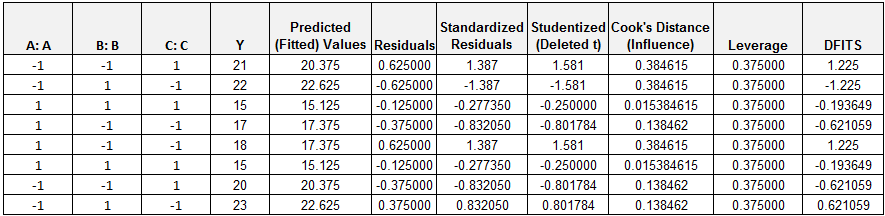1. Select the entire range of the residuals in the residual report
2. Click SigmaXL -> Graphical Tools -> Histograms & Descriptive Statistics
3. A new window named “Histograms & Descriptive” appears and the selected range is automatically populated into the box below “Please select your data”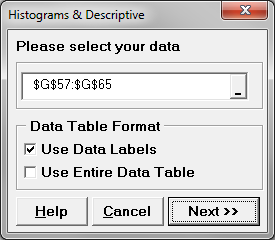4. Click “Next>>”
5. A new window named “Histograms & Descriptive Statistics” pops up.
6. Select “Residuals” as the “Numeric Data Variables (Y)”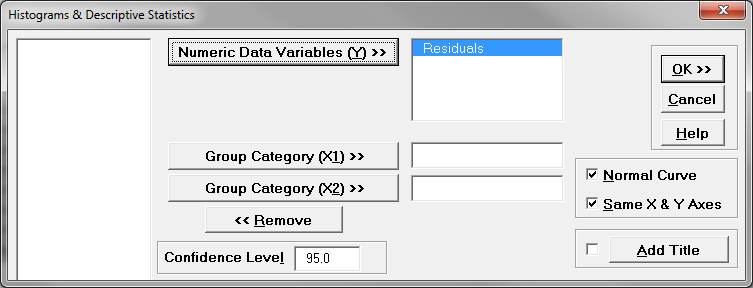7. Click “OK>>”
8. The histogram and the normality test of the residuals appear in the newly generated tab “Hist Descript (1)”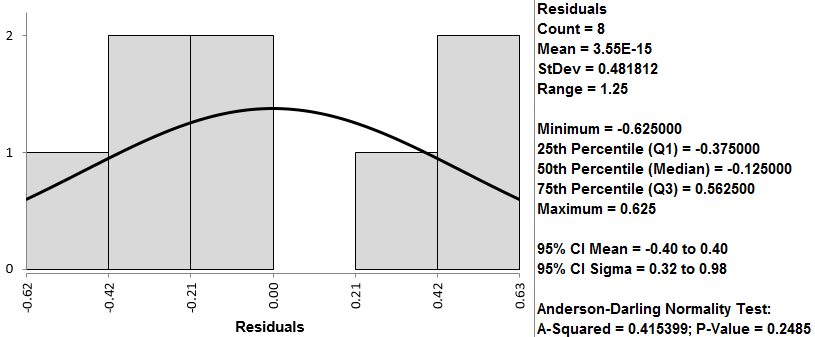If the p-value of the normality test is greater than the alpha level (0.05), the residuals are normally distributed. The p-value of the normality test is larger than alpha level (0.05). The residuals are normally distributed. Residuals’ mean is zero.

Step 7: Check whether the residuals are independent.

1. Select the entire range of the residuals in the residual report.
2. Click SigmaXL -> Control Charts -> Individuals & Moving Range
3. A new window named “Individuals and Moving Range” appears with the selected range automatically populated into the box below “Please select your data”.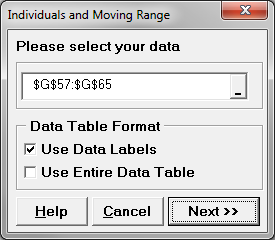4. Click “Next>>”
5. A new window named “Individuals and Moving Range Chart” pops up.
6. Select the “Residuals” as the “Numeric Data Variables (Y)”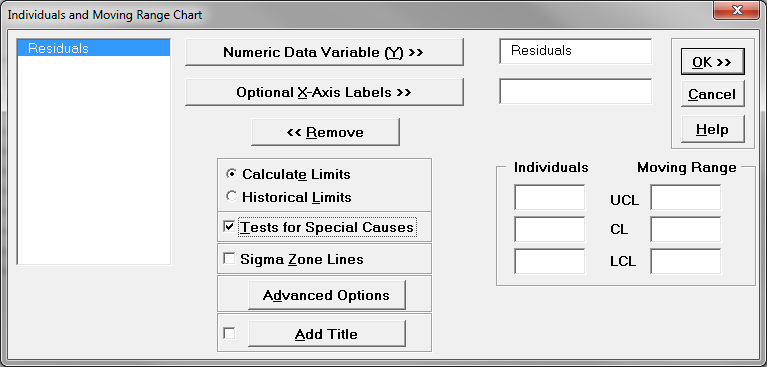7. Click “OK>>”
8. The control charts appear in the newly generated tab “Indiv & MR Charts (1)”.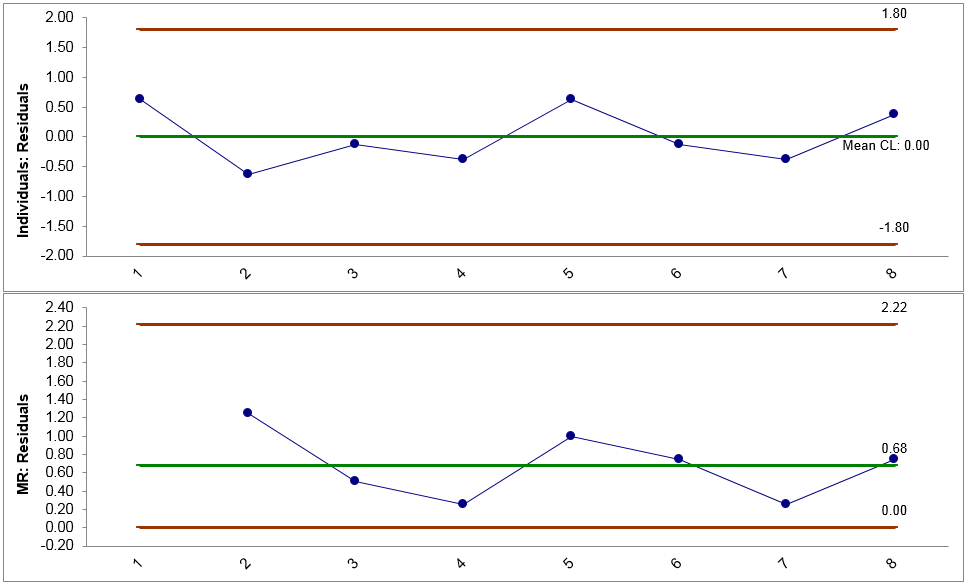If no data points on the control charts fail any tests, the residuals are in control and independent of each other. Note: The prerequisite of plotting IR chart for residuals: the residuals are in the time order. These are the control charts for estimating whether residuals are independent.

Step 8: Check whether the residuals have equal variance across the predicted response values. Close to the bottom of the tab “Analyze – 3 Factor DOE (1)” is the residual by predicted plot.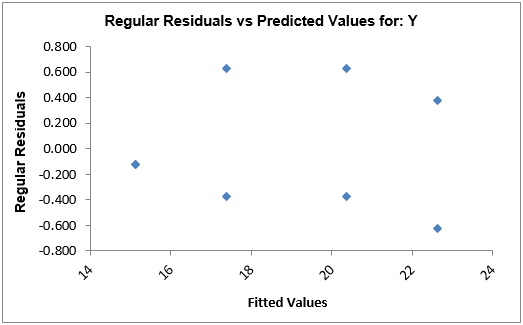Model summary: We look for patterns in which the residuals tend to have even variation across the entire range of the fitted response values.